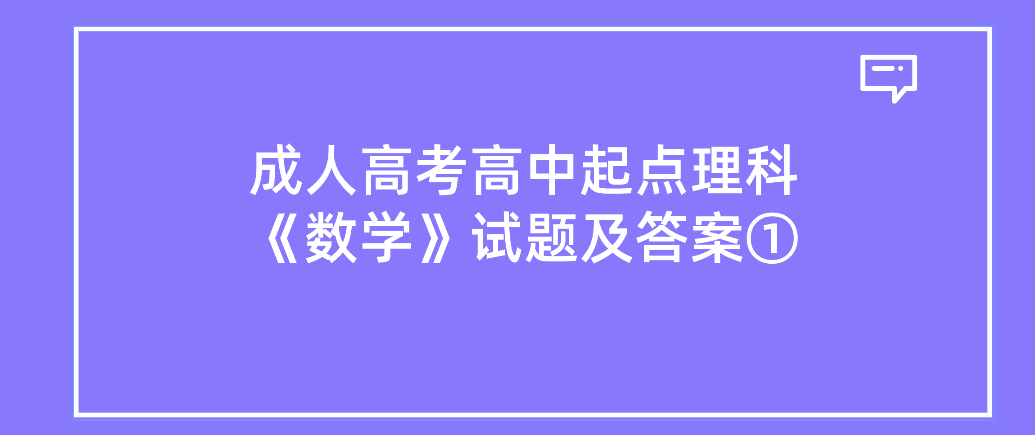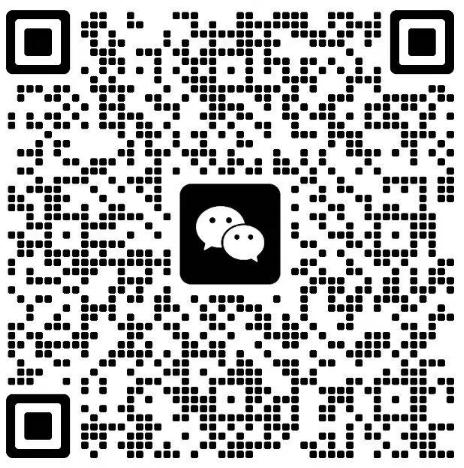# 2022年浙江成人高考高中起点理科《数学》试题及答案①1.设集合A={x|x=1},B={x|x3=1},则ANB=()

A.0

B.{1}

c.{-1}

D.{-1,1)

A={x|x3=1}={-11},B={x|x=1}={1},A0B={1}.

2、函数()-1]=lg的定义域是()

A.(0,+x0)

B.(-xc,0)

c.(0,1)

D.(1,+xc)

3.下列函数的图像与y=f(x)的图像关于原点对称的是()

A.y=-f(x)

B.y=f(-x)

Cy=-f(-x)

D.y=|f(x)

4.下列通数中，在区间(0,+xc)上是增函数的是()

A.y=-x

B.y=x-2

c.y=()

D.y=log:

B项中y=2x,当x>0时，y>0,故y=x-2在(0,+x)为增函数。

5.直线3x+y-2=0经过()

A.x一I

B.第一、二、四象限

C.第一、二、三象限

D.第二、三、四象限

2023年全国成人高考还有

### 浙江成考网在线客服